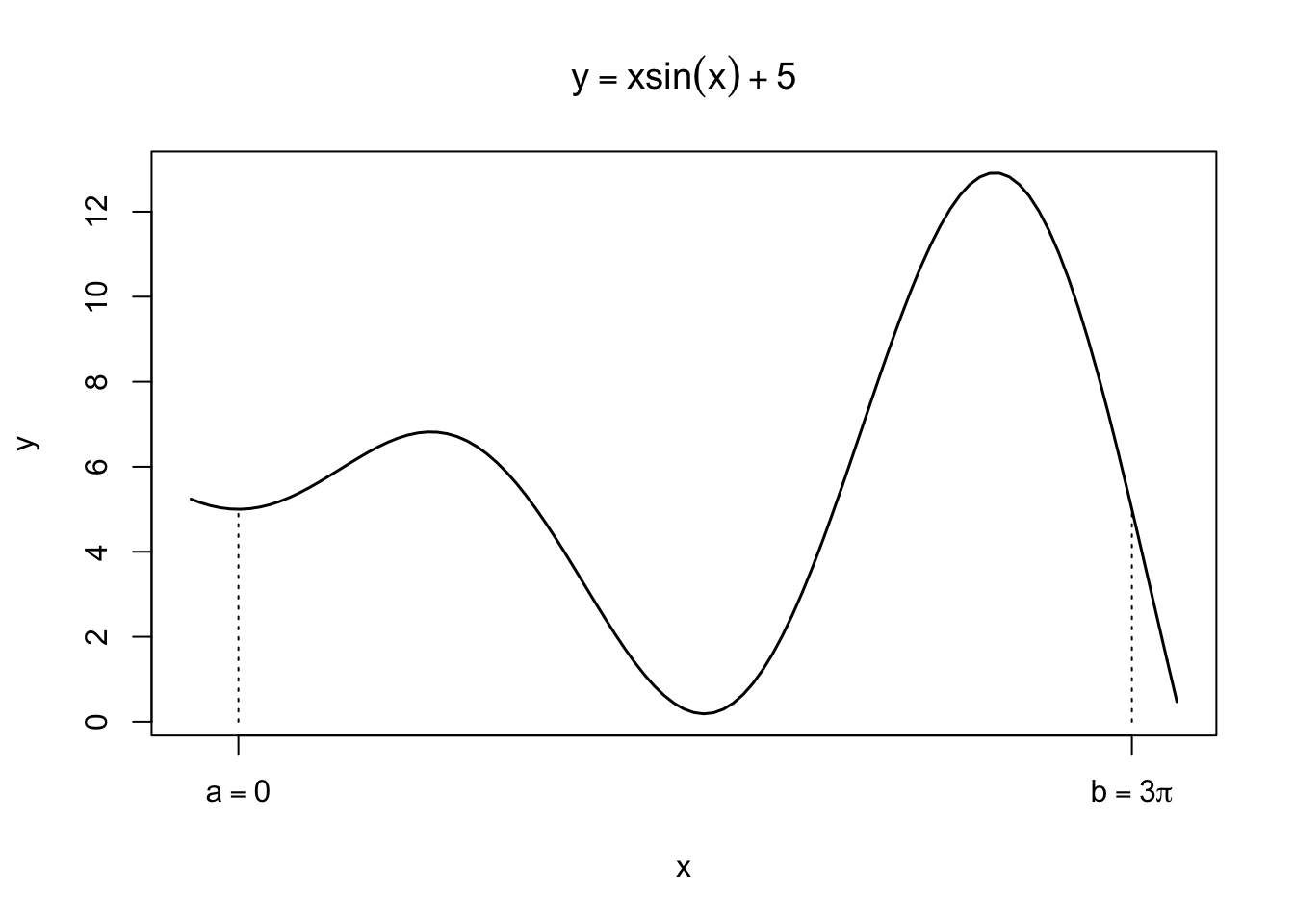# Introduction

## Monte Carlo

Monte Carlo method is a class of algorithms that draw random numbers and compute their statistics to approximate quantities of interest. The method uses randomness even to solve deterministic problems, for which deterministic algorithms are too inefficient to be practical. Stanislaw Ulam was one of the contributors who developed the method in the mid 20th Century. The method was named after Ulam’s uncle who just had to go Monte Carlo to gamble. Monte Carlo integration is the most common application of the method.

## Key ideas

Recall that, for many functions, it is impossible to carry out analytical integration, which is the motivation for Numerical Integration. Deterministic methods such as the trapezoidal rule and Simpson’s rule have their pros and cons. They often work pretty well in one-dimensional problems, but their performance rapidly deteriorates as the dimensionality increases. Monte Carlo (MC) integration is an alternative approach to high-dimensional problems, in which MC integration tends to be computationally more efficient than deterministic methods.

The theoretical foundation of MC integration is the law of large number. In words, for reasonable $$X$$ and $$h$$, the sample average converges to the true mean. Or, $\frac{1}{n}\sum_{i=1}^n h(X_i) \to \mathbb{E}h(X)$ as $$n\to\infty$$. How can this help with difficult integration?

Remember that, for continuous $$X$$, the expectation of $$h(X)$$ is defined as an integral: $\mathbb{E}h(X) = \int_{\mathcal{S}} h(x)f(x)dx ,$ where $$\mathcal{S}$$ is the support of $$X$$. Therefore, expressing a difficult integral in terms of the expectation of some random variable $$X$$, for which we can collect lots of samples, we can use the sample average to approximate that integral.

“we can collect lots of samples” is a catch and addressed in Sampling Methods. But, for now, let’s assume we can.

Suppose we want to integrate $$\phi(x)$$ over the range $$\mathcal{X}$$. Let $$f$$ be the PDF for $$X$$ such that $$\mathcal{X}\subseteq\mathcal{S}$$. Then,

\begin{align} \int_\mathcal{X}\phi(x)dx &= \int_\mathcal{S}\phi(x)\mathbb{1}_{\mathcal{X}}(x)dx\\ &= \int_\mathcal{S} \frac{\phi(x)\mathbb{1}_{\mathcal{X}}(x)}{f(x)}f(x)dx\\ &= \mathbb{E}\left[\frac{\phi(X)\mathbb{1}_{\mathcal{X}}(X)}{f(X)}\right] \end{align}

We can approximate this expectation by the sample mean estimator $$\hat{M}$$: $\hat{M} = \frac{1}{n}\sum_{i=1}^n \frac{\phi(X_i)\mathbb{1}_{\mathcal{X}}(X_i)}{f(X_i)} \approx \mathbb{E}\left[\frac{\phi(X)\mathbb{1}_{\mathcal{X}}(X)}{f(X)}\right].$

Note that $$\hat{M}$$ is an unbiased estimator, meaning $\mathbb{E}\hat{M} = \mathbb{E}\left[\frac{\phi(X)\mathbb{1}_{\mathcal{X}}(X)}{f(X)}\right].$

If $$\mathcal{X}=\mathcal{S}$$, of course, we can drop $$\mathbb{1}_{\mathcal{X}}$$ everywhere and have cleaner expressions.

As you may notice, in a sense, the indicator function $$\mathbb{1}_{\mathcal{X}}(x)$$ checks $$x$$’s membership in $$\mathcal{X}$$ — a member $$(1)$$ or not $$(0)$$. Judicious use of indicator function is powerful, allowing us to formulate many different quantities as expectations. Even probability can be expressed as an expectation: $P(X\in \mathcal{X}) = \int_\mathcal{X}f(x)dx = \int_{\mathcal{S}} \mathbb{1}_{\mathcal{X}}(x)f(x)dx = \mathbb{E}\mathbb{1}_{\mathcal{X}}(X).$

# Inferior performance in 1-D

Let’s compare MC integration with Simpson’s rule. A test problem is to integrate $\phi(x) = x\sin(x)+5 \enspace\text{over}\enspace \mathcal{X}=[0,3\pi],$ for which we know the answer is $$18\pi \approx 56.5486678$$. With only $$n=100$$ equally-spaced evaluation points, Simpson’s rule achieves $$56.5486719$$.In principle, we may use any continuous distribution provided $$\mathcal{X}\subseteq\mathcal{S}$$. We experiment here $$X_1\sim \text{U}(0,10)$$ and $$X_2\sim\text{exp}(1)$$ with $$n=10^4$$.

First, $$X_1\sim \text{U}(0,10)$$ has the support $$\mathcal{S}_1=[0,10]$$ and the PDF $$f_1(x)=1/10$$ for $$x\in\mathcal{S}_1$$ and $$0$$ otherwise. Hence, the estimator is $\hat{M}_1 = \frac{1}{n}\sum_{i=1}^n \frac{\phi(X_{1i})\mathbb{1}_{\mathcal{X}}(X_{1i})}{f_1(X_{1i})} = \frac{1}{n}\sum_{i=1}^n 10\phi(X_{1i})\mathbb{1}_{\mathcal{X}}(X_{1i}).$

Next, $$X_2\sim\text{exp}(1)$$ has the support $$\mathcal{S}_2=[0,\infty)$$ and the PDF $$f_2(x)=e^{-x}$$ for $$x\in\mathcal{S}_2$$ and $$0$$ otherwise. Hence, the estimator is $\hat{M}_2 = \frac{1}{n}\sum_{i=1}^n \frac{\phi(X_{2i})\mathbb{1}_{\mathcal{X}}(X_{2i})}{f_2(X_{2i})} = \frac{1}{n}\sum_{i=1}^n e^{x}\phi(X_{2i})\mathbb{1}_{\mathcal{X}}(X_{2i}).$ Here are results.

# X1
x <- runif(n, 0, 10)
x <- x[x < 3*pi] # those in the integration range
10*sum(phi(x))/n
##  56.25112
# X2
x <- rexp(n)
x <- x[x < 3*pi] # those in the integration range
sum(exp(x)*phi(x))/n
##  40.01199

As you can see, the results tend to be inferior even with $$n=10^{4}$$ samples. In particular, $$\hat{M}_2$$ suffers high variance.

m <- 100
re <- rep(0,m)
for (i in 1:m) {
x <- rexp(n)
x <- x[x < 3*pi] # those in the integration range
re[i] <- sum(exp(x)*phi(x))/n
}
paste("Standard deviation =", round(sd(re),2))
##  "Standard deviation = 10.6"

Well, this is expected; what’s the point of using $$X$$ with the support of $$[0,\infty)$$ to compute the integral of $$\phi(x)$$ over $$[0,3\pi]$$? Moreover, implied by $$P(X_2\leq \pi)\approx 0.96$$ most samples are taken from $$[0,\pi]$$, and hence the integrand is rarely evaluated over $$[\pi,3\pi]$$. In cases like this, we cannot expect good approximation via MC integration. We will come back to this issue, inefficiency in particular, to motivate Importance sampling.

# Visual demos

To simplify problems and highlight key features, let’s consider the uniform distribution over some rectangle $$\mathcal{S}=[x_1,x_2]\times[y_1,y_2]\subset\mathbb{R}^2$$. Recall that the uniform distribution over $$\mathcal{S}\in\mathbb{R}^d$$ is equivalent to having the following PDF of $$X$$ $f(x) = \begin{cases} \frac{1}{|\mathcal{S}|} & x\in\mathcal{S}\\ 0 & \text{otherwise} \end{cases}$ where $$|\mathcal{S}| = \int_\mathcal{S}1dx$$, the area/volume of $$\mathcal{S}$$. It is a normalisation constant to make the PDF integrates to $$1$$.

## Estimating $$\pi$$

A canonical problem is to use MC integration to estimate $$\pi$$. First, define $\mathcal{X} = \{x\in\mathbb{R}^2 : \|x\|\leq1, x\geq0\},$ a quarter disk in the positive orthant whose area is $$\pi/4$$. Using the support $$\mathcal{S} = [0,1]^2$$ with $$|\mathcal{S}|=1$$, we have \begin{align} \frac{\pi}{4} &= \int_\mathcal{X}1dx\\ &= \int_\mathcal{S} \mathbb{1}_\mathcal{X}(x)dx\\ &= \int_\mathcal{S} \frac{\mathbb{1}_\mathcal{X}(x)}{f(x)}f(x)dx\\ &= \int_\mathcal{S} \mathbb{1}_\mathcal{X}(x)f(x)dx\\ &= \mathbb{E}\left[\mathbb{1}_{\mathcal{X}}(X)\right] \end{align}

The idea is to use MC integration to approximate the last expectation, which in turn tells us $$\pi$$.

n <- 1e5
x <- c()
y <- c()
for (i in 1:n) {
xy <- runif(2)
if (sum(xy^2)<=1) {
x <- c(x, xy)
y <- c(y, xy)
}

if (i%%5e3 == 0) {
plot(x, y, xlim=c(0,1), ylim=c(0,1), ann=FALSE, pch=19, cex=0.1)
title(paste("n = ", i, ", ", "π = ", round(4*length(x)/i, 5), sep=""))
}
}